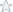# Math-133 (Unit 3)

Solve the following equations algebraically. You must show all your work.
Learn how to type math roots and fractions by clicking on the link in the assignment list. Alternately, you may type
x^(1/3) as cuberoot(x) and show raising to the nth power as ^n, like x 3 is typed x^3.

What potential solution did you obtain? Explain why this is this not a solution.
3) The volume of a cube is given by V = s3, where s is the length of a side. Find the length of a side of a cube(round the answer to three decimal places) if the volume is
a) 800 cm3.

b) 500 cm3.

The formula to find the wind chill temperature is given by
Where,
W is Wind Chill temperature (temperature with no wind)
T is actual temperature in Celcius

V is wind speeds in m/sec
Find the Wind Chill temperature given the following:

a) T = 10, v = 9m/sec
b) T = 0 , v = 15m/sec
c)T = -10, v = 20m/sec

Detailed solutions with work shown.

Question Files
MATH133_Unit 3 IP2.pdf

Subjects: Algebra
Topic: Indiviual Project-C
Level: College/University
Tags:

### Algebra,Math-Unit 3

Price\$5.95

Math Genius
 Manny I. Member Since: Aug 2007 Customer Rating: 92.8 Projects Completed: 487 Total Earnings: Private +1 Ratings from clients: 89
Project Details
Question Files
MATH133_Unit 3 IP2.pdf

Subjects: Algebra
Topic: Indiviual Project-C
Level: College/University
Tags:

## Algebra,Math-Unit 3

Customer Reviews
Rated 15 Times
Rating( 2.5 / 5 Stars)

## Reviews of the solutions left by other users

Review By: ()

1 stars
on
Comments: "More details needed!!!!! Waste of money!"

Not exactly what you are looking for?
We regularly update our math homework solutions library and are continually in the process of adding more samples and complete homework solution sets. If you do not find what you are looking for, just go ahead and place an order for a custom created homework solution. You can hire/pay a math genius to do your homework for you exactly to your specifications.